# PEMDAS

Go back to  'Numbers'

 1 Introduction to PEMDAS 2 What is PEMDAS? 3 When to use PEMDAS? 4 Important Notes on PEMDAS 5 Solved Examples on PEMDAS 6 Common Mistakes 7 Thinking out of the Box! 8 Practice Questions on PEMDAS 9 Important Topics of PEMDAS 10 Maths Qlympiad Sample Papers 11 Frequently Asked Questions (FAQs)

We at Cuemath believe that Math is a life skill. Our Math Experts focus on the “Why” behind the “What.” Students can explore from a huge range of interactive worksheets, visuals, simulations, practice tests, and more to understand a concept in depth.

Book a FREE trial class today! and experience Cuemath's LIVE Online Class with your child.

## Introduction to PEMDAS

There are different scenarios where everything goes through various steps in a fixed sequence.

Consider the following scenario.

Ryan and Ruhi visited a toy factory.

They both observed the processes followed in the factory to manufacture toys.

Toys are first designed.

Next, they are built and packed in boxes.

Finally, they are checked for quality before being shipped to stores.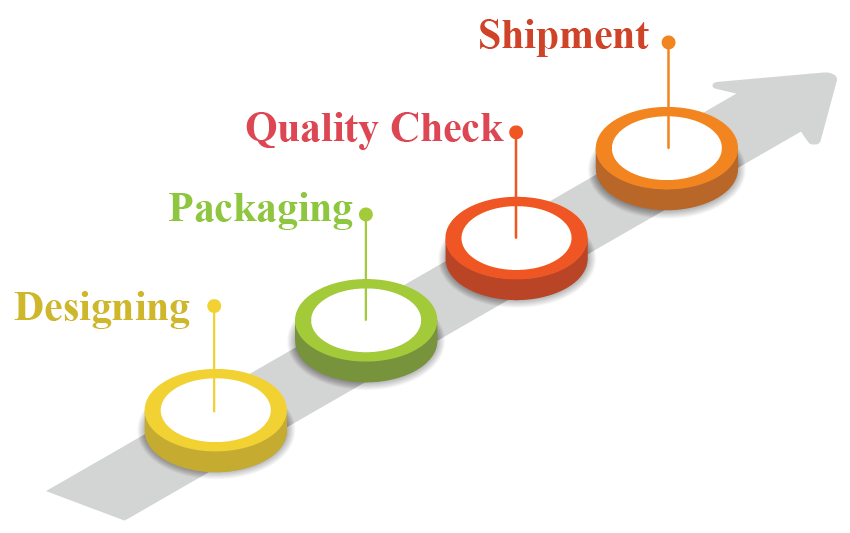Everything is done in a set order.

Similarly, arithmetic operations are done in a well-ordered manner.

Let us learn the order of operations in Mathematics.

The symbols shown below are the operators.

These are used to perform the mathematical operations on the numbers.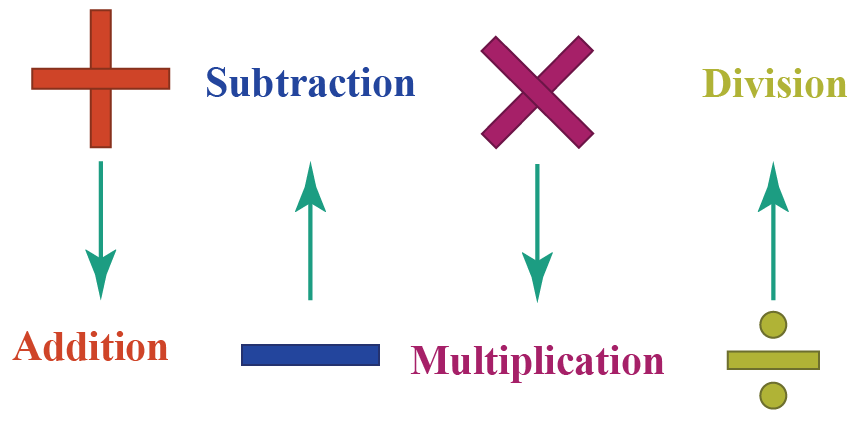Finding the answer to mathematical operations is fairly simple when only one operator is involved.

What if multiple operators are involved?

This could get a little trickier! Let’s see how.

Ryan and Ruhi solved a mathematical expression $$5+2 \times 3$$ separately.

This is how they solved it.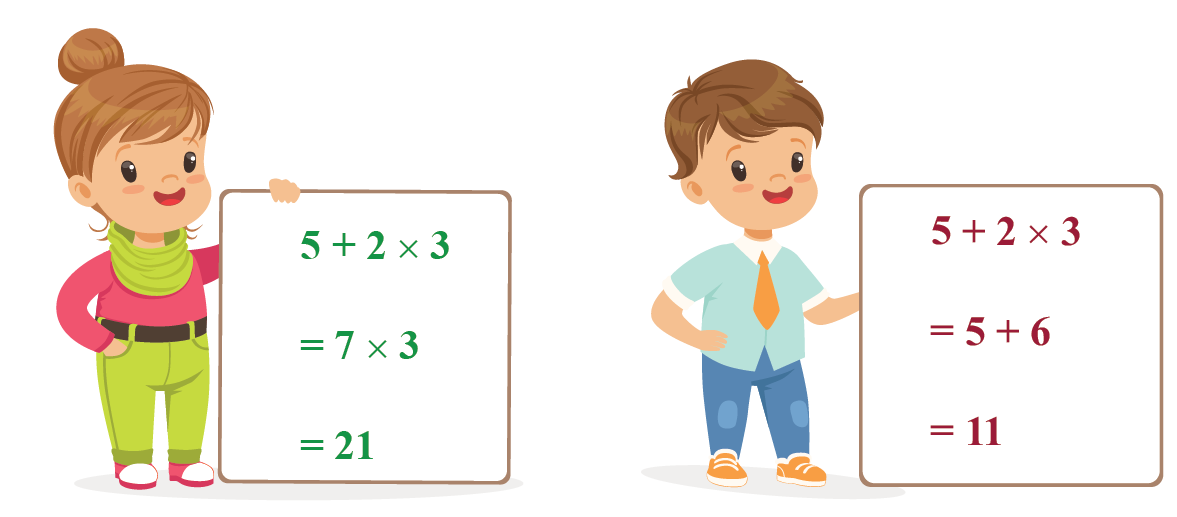As you can observe, Ryan and Ruhi got different answers.

There can only be one correct answer to this expression in mathematics!

Can you decide who is correct?

## What is PEMDAS?

### PEMDAS: Definition

PEMDAS is a set of rules which are followed while solving mathematical expressions.

This rule starts with Parentheses, and then operations are performed on the exponents or powers.

Next, we perform operations on multiplication or division from left to right.

Finally, operations on addition or subtraction are performed from left to right.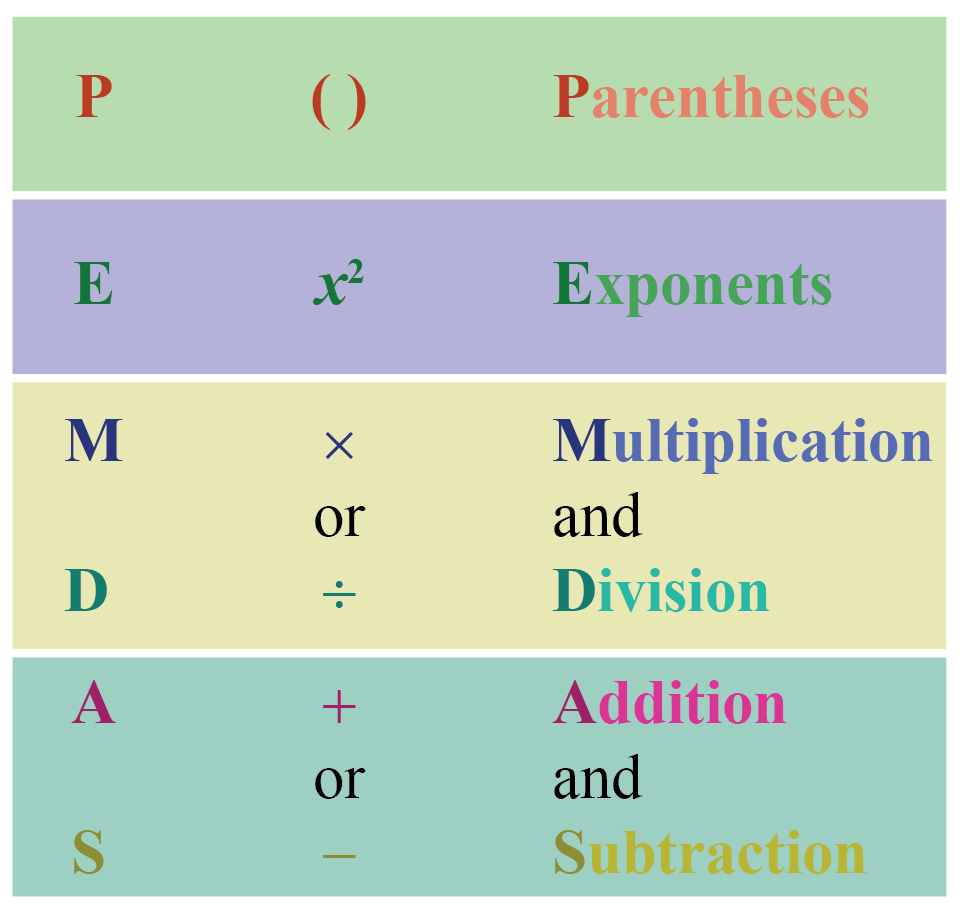If you stick to this order of operations in the PEMDAS rule, you will always get the correct answer.Let us understand PEMDAS with the help of an example.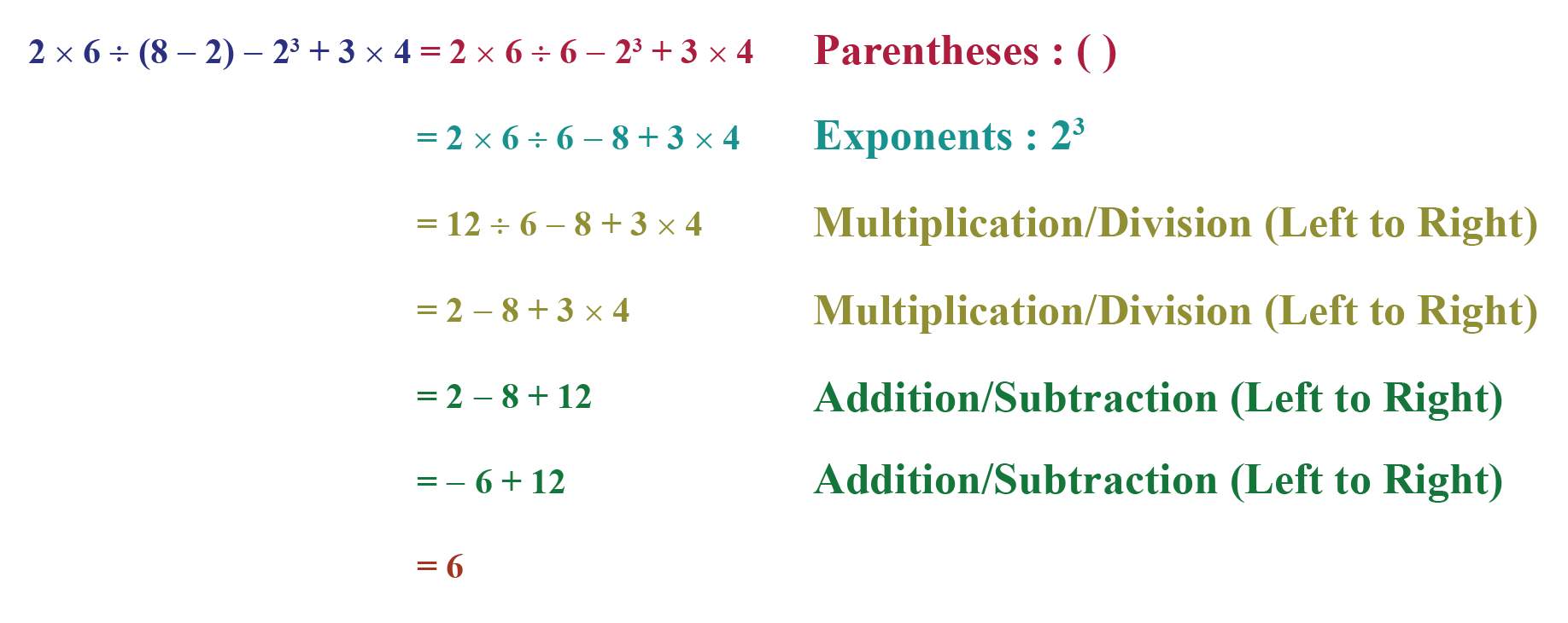The PEMDAS rule is similar to the BODMAS rule.

There is a difference in the name of the rule because certain terms are known by different names in different locations.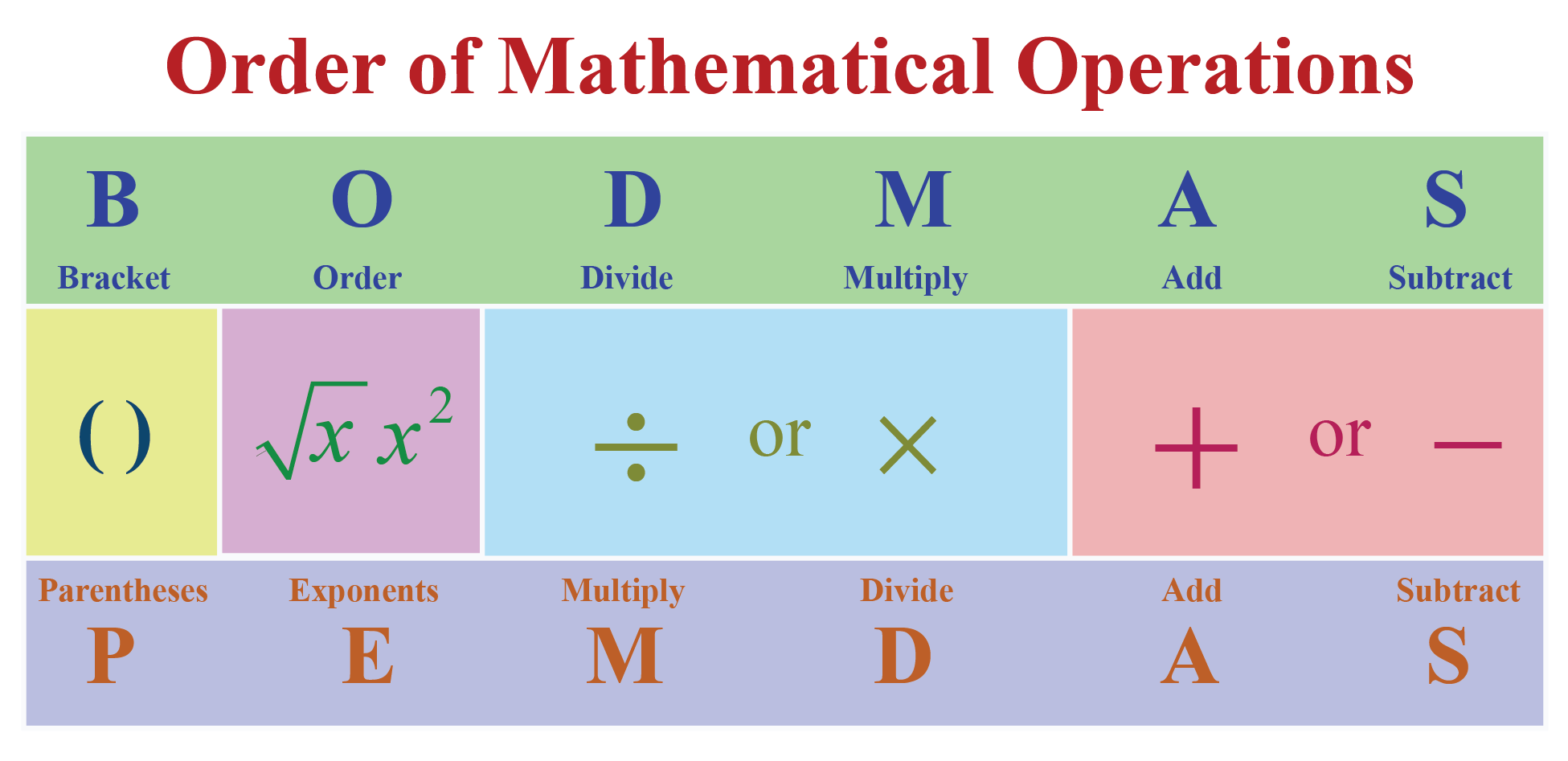## When to use PEMDAS?

When there is more than one operation in a mathematical expression, we use the PEMDAS method.

PEMDAS in Math gives you a proper structure to produce a unique answer for every mathematical expression.

There is a sequence of certain rules that need to be followed when using the PEMDAS method.

Once you get the hang of these rules, you can do multiple steps at once.

 Example 1

$$18\div(8-2\times 3)$$

Solution:

\begin{align}18\div(8-2\times 3)&=18\div(8-6)\\&=18\div 2\\&=9\end{align}

 $$18\div(8-2\times 3)=9$$
 Example 2

$$(4\times 3\div 6+1)\times 3^{2}$$

Solution:

\begin{align}(4\times 3\div 6+1)\times 3^{2}&=(12\div 6+1)\times 3^{2}\\&=(2+1)\times 3^{2}\\&=3 \times 3^{2}\\&=3 \times 9\\&=27\end{align}

 $$(4\times 3\div 6+1)\times 3^{2}=27$$
 Example 3

$$4^{2}\div 2+4\times 3$$

Solution:

\begin{align}4^{2}\div 2+4\times 3&=16\div 2+4\times 3\\&=8+12\\&=20\end{align}

 $$4^{2}\div 2+4\times 3=20$$

Practicing these questions might have made you understand that the order of operations in the PEMDAS rule is nothing but the order of operations that we use to solve difficult mathematical expressions.

Now, let us get back to the mathematical expression given to Ruhi and Ryan to solve, and decide who got the correct answer.

\begin{align}5+2 \times 3&=5+6\\&=11\end{align}

Thus, based on the order of operations, Ryan got the correct answer.

But, where did Ruhi go wrong? Let’s see.

According to the PEMDAS rule, addition is followed after multiplication.

But, Ruhi performed addition before multiplication.

This step resulted in an incorrect answer.

## Common Mistakes while using PEMDAS rule in Math

The presence of multiple brackets usually causes confusion.

If we don't know which bracket to solve first, it could lead to an incorrect answer.

We will now learn how to solve this expression with multiple brackets.

$4 + 3[8 –2(6 – 3)] \div 2$

We will begin with working from the inside of the brackets.

We will solve the inner-most bracket first and then move outside.

Starting with $$6 – 3 = 3$$, we get:

$4 + 3[8 – 2(3)] \div 2$

Next, multiplying $$2 (3)=6$$ or $$2 \times 3=6$$, we get:

$4 + 3[8 – 6] \div 2$

There is one bracket left, $$[8 – 6] = 2$$

$4 + 3 \div 2$

Solving $$3 $$ or $$3 \times 2 = 6$$, we have:

$4 + 6 \div 2$

We can observe that all the expressions in the brackets are solved.

Based on PEMDAS, we know that division comes next, hence, $$6 \div 2 = 3$$

$4 + 3$

And lastly, addition $$4 + 3 = 7$$

 $$4 + 3[8 –2(6 – 3)] \div 2=7$$Think Tank
1. Simplify the following expression using the order of operations.
$4\times(18\div{2+1-8\div4})$

CLUEless in Math? Check out how CUEMATH Teachers will explain PEMDAS to your kid using interactive simulations & worksheets so they never have to memorise anything in Math again!

Explore Cuemath Live, Interactive & Personalised Online Classes to make your kid a Math Expert. Book a FREE trial class today!

## Practice Questions

Here are a few activities for you to practice.

## Important Topics

Given below are the list of topics that are closely connected to PEMDAS. These topics will also give you a glimpse of how such concepts are covered in Cuemath.

IMO (International Maths Olympiad) is a competitive exam in Mathematics conducted annually for school students. It encourages children to develop their math solving skills from a competition perspective.

## 1. What is the rule for PEMDAS?

The PEMDAS rule gives us the correct sequence for solving a mathematical expression.

In PEMDAS rule, operations are performed in parentheses first.

Next, operations are performed on exponents or powers.

This is followed by the operations on multiplication or division from left to right, whichever comes first.

Finally,the operations on addition or subtraction are performed from left to right, whichever comes first.

## 2. Do you multiply or divide first in PEMDAS?

In PEMDAS rule, we solve operations on multiplication and division from left to right.

We perform the operation that comes first.

## 3. When do we apply the PEMDAS rule?

PEMDAS Rule is applied for solving difficult mathematical expression involving more than one operation like addition, subtraction, multiplication or division.

More Important Topics
Numbers
Algebra
Geometry
Measurement
Money
Data
Trigonometry
Calculus